Anzeige

23. Dec 2022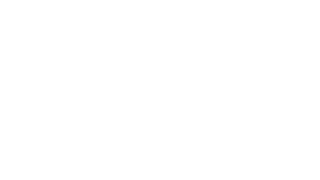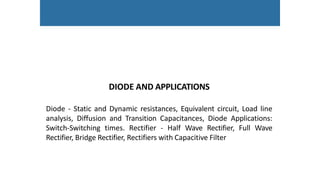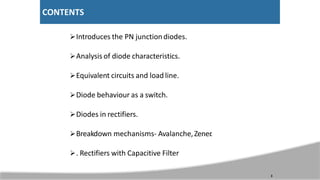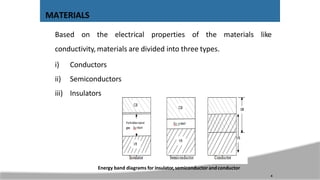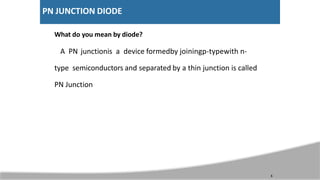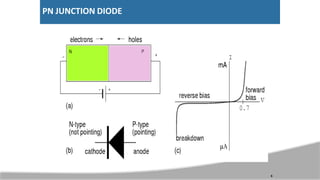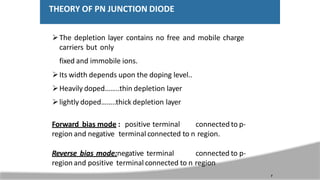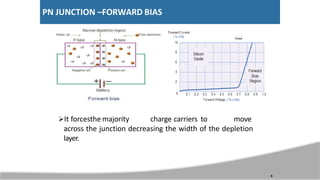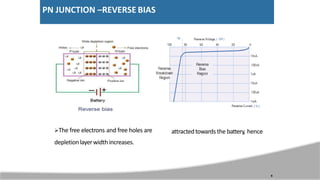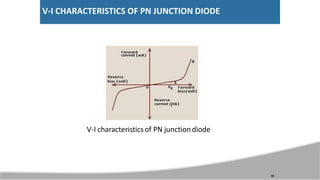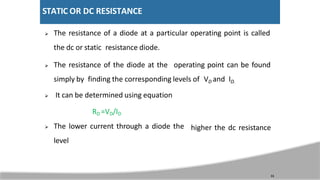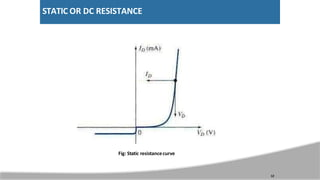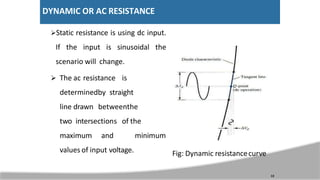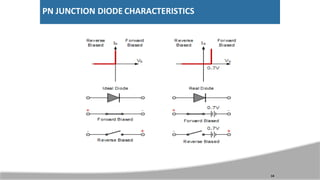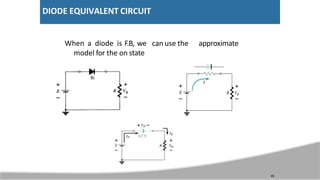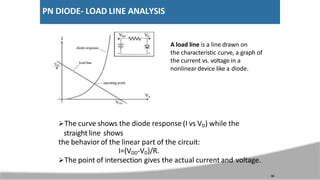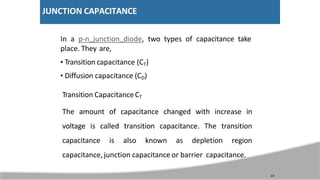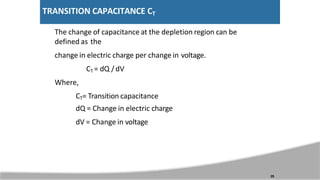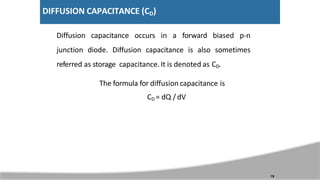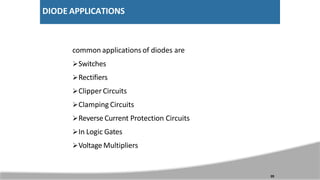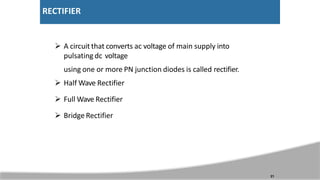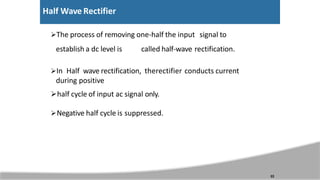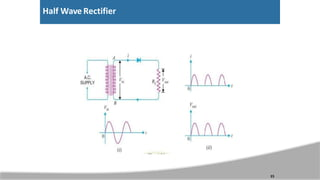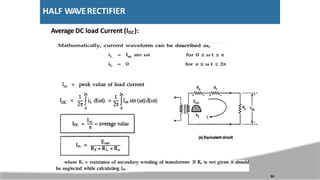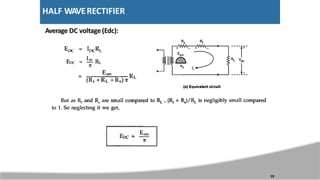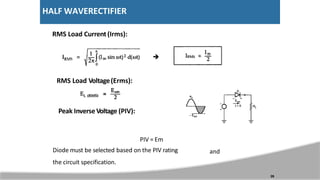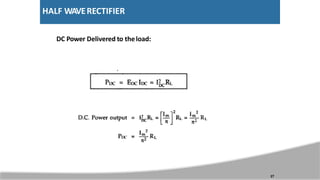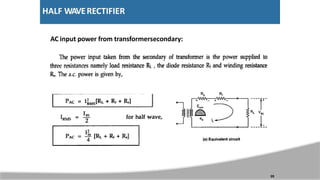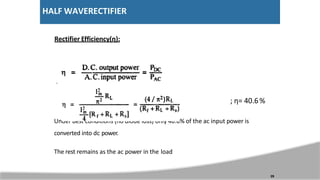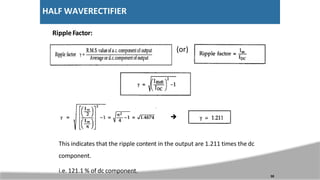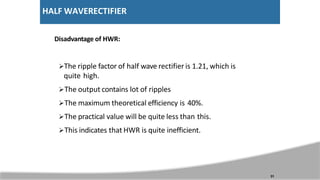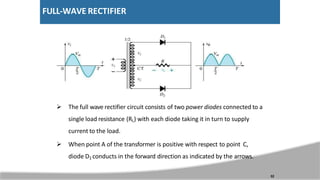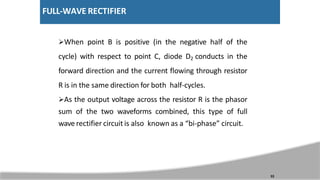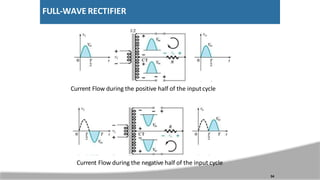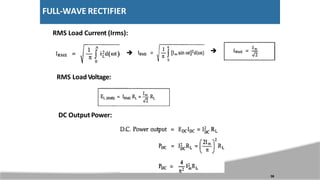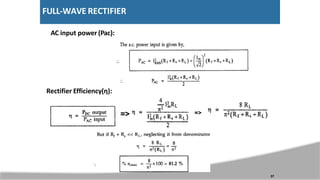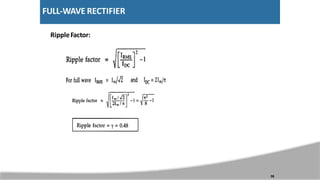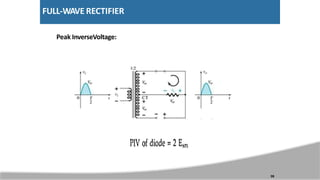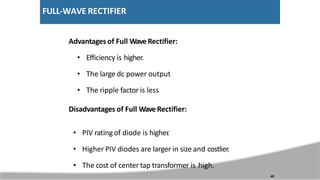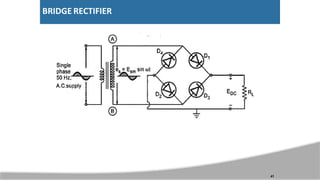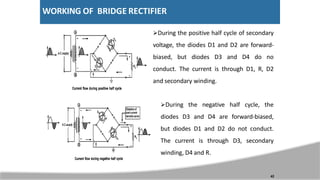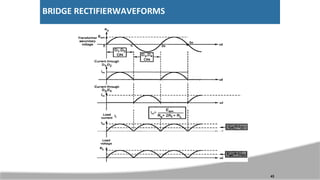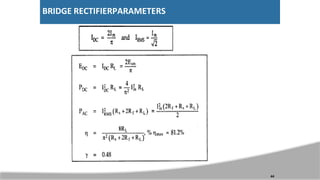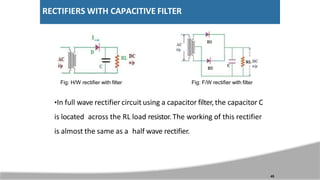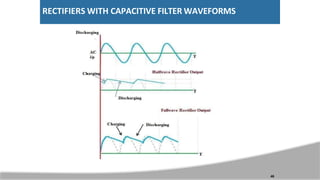1 von 46
Anzeige

1. DIODE AND APPLICATIONS Diode - Static and Dynamic resistances, Equivalent circuit, Load line analysis, Diffusion and Transition Capacitances, Diode Applications: Switch-Switching times. Rectifier - Half Wave Rectifier, Full Wave Rectifier, Bridge Rectifier, Rectifiers with Capacitive Filter
2. CONTENTS Introduces the PN junctiondiodes. Analysis of diode characteristics. Equivalent circuits and load line. Diode behaviour as a switch. Diodes in rectifiers. Breakdown mechanisms- Avalanche,Zener . . Rectifiers with Capacitive Filter 3
3. Based on the electrical properties of the materials like conductivity, materials are divided into three types. i) Conductors ii) Semiconductors iii) Insulators Energy band diagrams for insulator, semiconductor andconductor MATERIALS 4
4. What do you mean by diode? A PN junctionis a device formedby joiningp-typewith n- type semiconductors and separated by a thin junction is called PN Junction PN JUNCTION DIODE 5
5. PN JUNCTION DIODE 6
6. THEORY OF PN JUNCTION DIODE The depletion layer contains no free and mobile charge carriers but only fixed and immobile ions. Its width depends upon the doping level.. Heavily doped……..thin depletion layer lightly doped……..thick depletion layer Forward bias mode : positive terminal connected to p- region and negative terminal connected to n region. Reverse bias mode:negative terminal connected to p- region and positive terminal connected to n region 7
7. PN JUNCTION –FORWARD BIAS It forcesthe majority charge carriers to move across the junction decreasing the width of the depletion layer. 8
8. attractedtowards the battery, hence The free electrons and free holes are depletionlayerwidthincreases. PN JUNCTION –REVERSE BIAS 9
9. V-I CHARACTERISTICS OF PN JUNCTION DIODE V-I characteristics of PN junctiondiode 10
10. STATIC OR DC RESISTANCE  The resistance of a diode at a particular operating point is called the dc or static resistance diode.  The resistance of the diode at the operating point can be found simply by finding the corresponding levels of VD and ID.  It can be determined using equation 11 RD =VD/ID  The lower current through a diode the level higher the dc resistance
11. STATIC OR DC RESISTANCE Fig: Static resistancecurve 12
12. DYNAMIC OR AC RESISTANCE Static resistance is using dc input. If the input is sinusoidal the scenario will change.  The ac resistance is determinedby straight line drawn betweenthe two intersections of the maximum and minimum values of input voltage. Fig: Dynamic resistancecurve 13
13. PN JUNCTION DIODE CHARACTERISTICS 14
14. DIODE EQUIVALENT CIRCUIT approximate When a diode is F.B, we can use the model for the on state 15
15. PN DIODE- LOAD LINE ANALYSIS The curve shows the diode response (I vs VD) while the straight line shows the behavior of the linear part of the circuit: I=(VDD-VD)/R. The point of intersection gives the actual current and voltage. 16 A load line is a line drawn on the characteristic curve, a graph of the current vs. voltage in a nonlinear device like a diode.
16. JUNCTION CAPACITANCE In a p-n junction diode, two types of capacitance take place. They are, • Transition capacitance (CT) • Diffusion capacitance (CD) Transition CapacitanceCT The amount of capacitance changed with increase in voltage is called transition capacitance. The transition capacitance is also known as depletion region capacitance, junction capacitance or barrier capacitance. 17
17. TRANSITION CAPACITANCE CT The change of capacitance at the depletion region can be defined as the change in electric charge per change in voltage. CT = dQ /dV Where, CT= Transition capacitance dQ = Change in electric charge dV = Change in voltage 25
18. DIFFUSION CAPACITANCE (CD) Diffusion capacitance occurs in a forward biased p-n junction diode. Diffusion capacitance is also sometimes referred as storage capacitance. It is denoted as CD. The formula for diffusion capacitance is CD = dQ / dV 19
19. DIODE APPLICATIONS common applications of diodes are Switches Rectifiers Clipper Circuits Clamping Circuits Reverse Current Protection Circuits In Logic Gates Voltage Multipliers 20
20. RECTIFIER  A circuit that converts ac voltage of main supply into pulsating dc voltage using one or more PN junction diodes is called rectifier.  Half Wave Rectifier  Full Wave Rectifier  Bridge Rectifier 21
21. Half Wave Rectifier The process of removing one-half the input signal to establish a dc level is called half-wave rectification. In Half wave rectification, therectifier conducts current during positive half cycle of input ac signal only. Negative half cycle is suppressed. 22
22. Half Wave Rectifier 23
23. HALF WAVERECTIFIER Average DC load Current (IDC): 24
24. HALF WAVERECTIFIER Average DC voltage(Edc): 25
25. HALF WAVERECTIFIER RMS Load Current (Irms):  RMS Load Voltage(Erms): Peak Inverse Voltage (PIV): and 26 PIV = Em Diode must be selected based on the PIV rating the circuit specification.
26. HALF WAVERECTIFIER DC Power Delivered to theload: 27
27. HALF WAVERECTIFIER AC input power from transformersecondary: 28
28. HALF WAVERECTIFIER Rectifier Efficiency(η): ; η= 40.6% Under best conditions (no diode loss) only 40.6% of the ac input power is converted into dc power. The rest remains as the ac power in the load 29
29. HALF WAVERECTIFIER RippleFactor: (or) 30  This indicates that the ripple content in the output are 1.211 times the dc component. i.e. 121.1 % of dc component.
30. HALF WAVERECTIFIER Disadvantage of HWR: The ripple factor of half wave rectifier is 1.21, which is quite high. The output contains lot of ripples The maximum theoretical efficiency is 40%. The practical value will be quite less than this. This indicates that HWR is quite inefficient. 31
31. FULL-WAVE RECTIFIER  The full wave rectifier circuit consists of two power diodes connected to a single load resistance (RL) with each diode taking it in turn to supply current to the load.  When point A of the transformer is positive with respect to point C, diode D1 conducts in the forward direction as indicated by the arrows. 32
32. FULL-WAVE RECTIFIER When point B is positive (in the negative half of the cycle) with respect to point C, diode D2 conducts in the forward direction and the current flowing through resistor R is in the same direction for both half-cycles. As the output voltage across the resistor R is the phasor sum of the two waveforms combined, this type of full wave rectifier circuit is also known as a “bi-phase” circuit. 33
33. FULL-WAVE RECTIFIER Current Flow during the positive half of the inputcycle 34 Current Flow during the negative half of the input cycle
34. FULL-WAVE RECTIFIER Average DC current: Average (DC): Voltage 35
35. FULL-WAVE RECTIFIER RMS Load Current (Irms):   RMS LoadVoltage: DC Output Power: 36
36. FULL-WAVE RECTIFIER AC input power (Pac): Rectifier Efficiency(η): => => 37
37. FULL-WAVE RECTIFIER RippleFactor: 38
38. FULL-WAVE RECTIFIER Peak InverseVoltage: 39
39. FULL-WAVE RECTIFIER Advantages of Full WaveRectifier: • Efficiency is higher. • The large dc power output • The ripple factor is less Disadvantages of Full WaveRectifier: • PIV ratingof diode is higher . • Higher PIV diodes are larger in size and costlier. • The cost of center tap transformer is high. 40
40. BRIDGE RECTIFIER 41
41. WORKING OF BRIDGE RECTIFIER During the positive half cycle of secondary voltage, the diodes D1 and D2 are forward- biased, but diodes D3 and D4 do no conduct. The current is through D1, R, D2 and secondary winding. During the negative half cycle, the diodes D3 and D4 are forward-biased, but diodes D1 and D2 do not conduct. The current is through D3, secondary winding, D4 and R. 42
42. BRIDGE RECTIFIERWAVEFORMS 43
43. BRIDGE RECTIFIERPARAMETERS 44
44. RECTIFIERS WITH CAPACITIVE FILTER Fig: H/W rectifier with filter Fig: F/W rectifier with filter •In full wave rectifier circuit using a capacitor filter,the capacitor C is located across the RL load resistor. The working of this rectifier is almost the same as a half wave rectifier. 45
45. RECTIFIERS WITH CAPACITIVE FILTER WAVEFORMS 46
Anzeige